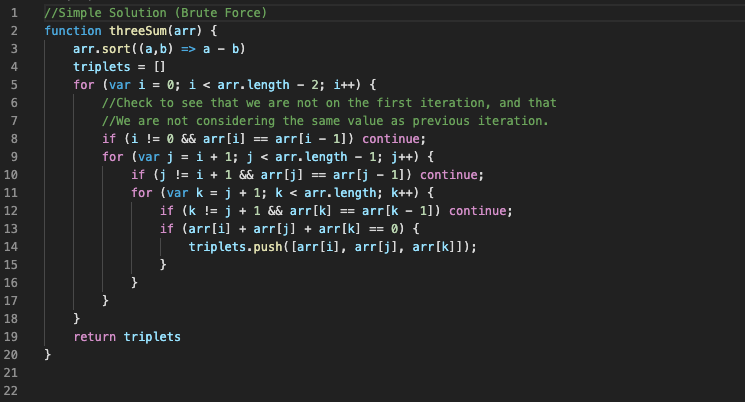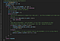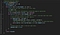# Three Ways to Solve 3Sum

An introduction to algorithms with a common interview problem

Introduction

Perhaps you are familiar with the 3Sum problem or a derivative of it. The problem is as follows: Given an array of n integers, find elements a, b, and c such that a + b + c = 0. Return all unique triplets that sum to 0. There are variations where you may be given a target sum instead of 0, or may need to find only one such triplet, but the process more or less remains the same:

Simple: Using 3 For Loops

For this method, we are going to start by sorting our input array, and then iterate through our array with 3 nested for loops (i, j, k), selecting triplets that sum to 0. To account for duplicates, we will make sure that we are not considering the same values for the same index variable twice. For example, if we have sorted array: [-4, -1, -1, 0, 2, 2, 3, 4, 5], we want to make sure that the second (or third, etc.) ‘-1’ will not be considered by our same index variable. When i == 1, sArr[i] will be -1, but when i == 2, we will skip to i == 3. Time complexity is cubic time: O(n3).Simple Solution

Intermediate: Using 2 Pointers

With the 2 pointers method, we are going to first sort our input array, and then iterate over the array from left to right with 1 for loop. At each iteration i, we check to see that our value at index i is not the same as the it was in the previous iteration, and then we will create left and right “pointer” variables, which are more or less index variables. The left pointer l will initialize at i + 1 (l = i + 1) and the right pointer r at the end of the array (r = arr.length — 1). Next, we will use l and r to check sets of values by doing the following: if arr[i] + arr[l] + arr[r] sums to 0, then we can add that triplet into our triplet array, increment l and decrement r. If the sum is less than 0, increment l, so that our next sum wil be larger, and thus closer to, if not exactly, 0, and if it is the sum is greater than 0, decrement r, so that our next sum will be smaller. Time complexity is quadratic time: O(n2).Intermediate Solution

Advanced: Hash

This solution is a bit more difficult and abstract than the first two. In the first solution, we employed brute force to find a solution. Pretty simple concept: check every set, and throw sets that fit criteria into output array. In the second solution, we used ‘pointers’ to conditionally iterate over the subArray sArr[i…]. Again, this was pretty straightforward. In the hash solution, we will add one more layer of abstraction. Consider the problem again, ignoring for a minute the duplicates condition. We need to find a, b, and c so that a + b + c = 0. First, let’s iterate over our array, and give a a value of A, which is simply sArr[i]. Now, our problem becomes b + c = -A. Next, we will initialize a hash, and immediately after, start iterating over the remaining array. Each iteration will give b a value B, which is sArr[j]. Our problem is now c = -A — B. If C, which is the result of the arithmetic, is in our hash, we will then add the set to our triplets array. Now, we account for duplicates by incrementing j until sArr[j] changes values. Finally, we tell our hash that we have seen the value at sArr[j]. Time complexity is quadratic time: O(n2).Advanced Solution

Conclusion:

The first solution, which uses brute force, is important to understand because it demonstrates a fundamental knowledge of a particular language as well as an ability to systematically solve a problem. The intermediate solution adds a layer of abstraction, and decreases runtime by a factor of n. In an interview, this solution is generally the one that will be expected, and works better for this problem than the hash solution. The hash solution would be ideal for a 2sum variant.